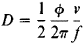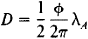Also found in: Acronyms.
The following article is from The Great Soviet Encyclopedia (1979). It might be outdated or ideologically biased.

a device for measuring distances by the velocity and transit time of radio waves transmitted along the line being measured and reflected back from the line’s end point. Distinctions are made between passive and active reflection in radio ranging, and between systems using pulsed signals and those using continuous signals.

In systems using passive reflection, two signals reach the receiver input—a direct signal from the radio transmitter and a delayed signal (relative to the direct signal) reflected from the object to which the distance is being measured. In pulsed systems, in which the radiated signal is a short radio pulse, an indicator measures the time delay T of the reflected pulse relative to the direct pulse; the distance thus measured is D= (½)VT, where v is the propagation velocity of the radio waves. In continuous systems, the radio signals used are varied periodically in frequency, and the indicator measures the frequency difference Ω between the direct and the reflected oscillations; the distance being measured is D = (ΩT/2 ∆f)v, where T is the period of the modulating oscillations, and ∆f is the frequency range of the modulation. Passive reflection is used in radar and radio altimeters.

In systems using active reflection, two stations—a master station and a slave station—are in operation at the ends of the line being measured. The radio signals may be pulsed or continuous, and they may have a single carrier frequency or have a frequency-modulated carrier. The radio signals received by the slave station are transformed and retransmitted. When continuous oscillations are used, the distance is measured by the phase method. If the signal used has a single carrier frequency f, the waves received by the slave station at the carrier frequency must be transformed into waves having a different oscillation frequency—one that is stiffly related to the frequency of the original oscillations by some ratio, such as 2/3 and 3/2; the waves are then retransmitted. In order to find the distance in this case, the indicator at the master station must measure the phase difference Φ between the radiated and received waves after an inverse transform of frequency; the distance measured will be equal toThe most accurate distance measurements (approximately 3 X 1O–6 times the measured distance) are achieved by systems that use modulated radio signals in the very-high-frequency ranges; the distance is measured by the phase shift of the modulating oscillations. In such systems, the master and slave stations radiate frequency-modulated or amplitude-modulated oscillations having the carrier frequencies fA and fB;in this case, fA= fB= fif, where fif is the intermediate frequency (if) at the station receivers. The frequency difference of the modulating oscillations of both stations FA—FB = ΔF is made low (of the order of 1000 hertz). The receivers at the stations do not have separate heterodynes; instead, oscillations from the station’s transmitters are used in mixers to shift the carrier frequency to the if. At the output of the receiver’s if amplifiers, if oscillations are obtained that are amplitude-modulated with sinusoidal oscillations of the low frequency ΔF. After detection at the slave station, these oscillations may be converted to pulses after detection, or they may modulate the oscillations of a subcarrier frequency and the signal thus obtained serves as an additional modulation for the radio transmitter. As a result, two low-frequency signals are produced at the receiver output of the master station, and their phase difference is measured by the indicator; the distance measured is given bywhere λA= v/FA is the wavelength of the modulating oscillations of the master station. In order to achieve high accuracy in measurements, λAD is chosen; this results in an ambiguity in the measurements, which is resolved by using several modulating oscillations at different frequencies. Systems based on the principle of active reflection are used in navigation and geodesy, and they have military applications.

REFERENCE

Pashchenkov, V. Z. Radio- i svetoda’nomery. Moscow, 1972.

I. L. Gill’